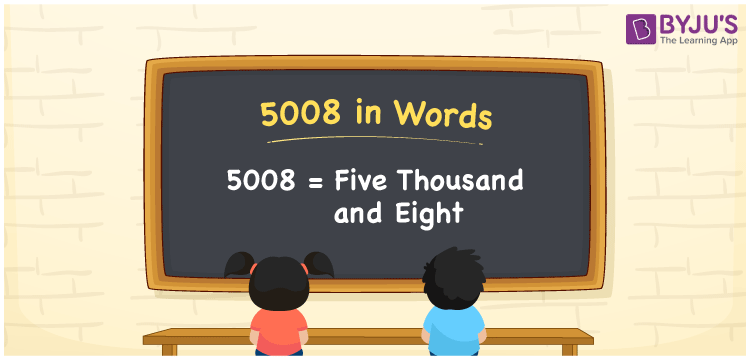# 5008 in Words

In English words, the number 5008 is expressed as “Five Thousand and Eight”. For writing the number 5008 in words, we use the place value system. Also, the number 5008 is a cardinal number. For instance, if you spent Rs. 5008 on purchasing books, you can write it as “ I spent Rs. Five thousand and Eight on purchasing books”.

 5008 in Words: Five Thousand and Eight. Five Thousand and Eight in Numerical Form: 5008.

## 5008 in English Words## How to Write 5008 in Words?

Below is the place value table for 5008, which is used to write the number 5008 in words.

 Thousands Hundreds Tens Ones 5 0 0 8

The expanded form of 5008 is as follows:

= 5 × Thousand + 0 × Hundred + 0 × Ten + 8 × One

= 5 × 1000 + 0 × 100 + 0 × 10 + 8 × 1

= 5000 + 8

= 5008

= Five thousand and eight

Hence, 5008 in words is five thousand and eight.

5008 in words – Five thousand and eight

Is 5008 an odd number? – No

Is 5008 an even number? – Yes

Is 5008 a perfect square number? – No

Is 5008 a perfect cube number? – No

Is 5008 a prime number? – No

Is 5008 a composite number? – Yes

## Frequently Asked Questions on 5008 in Words

Q1

### Write 5008 in words.

5008 in words is five thousand and eight.

Q2

### Simplify 5000 + 8, and express it in words.

Simplifying 5000 + 8, we get 5008. Hence, 5008 in words is five thousand and eight.

Q3

### Is 5008 a composite number?

Yes, 5008 is a composite number.